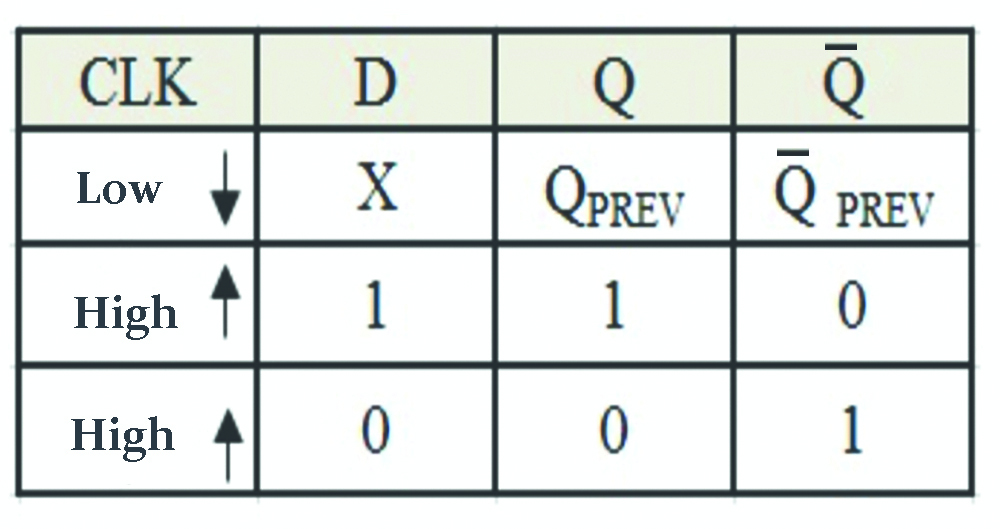# Data Flipflop (D-flipflop) || Sequential Logic || Bcis Notes

## Introduction

The Data flipflop is designed to overcome the problem of forbidden states and rest condition. It has single inputs that are inverted using NAND gates. The single input is called ‘D’ input. The ‘D’ flipflop is the modification of clock S-R flipflop.

In normal clock S-R flipflop, two separate inputs are required to SET and RESET the flipflop usually. This two-input are generated by two separate combinational circuits and may not be produced simultaneously. These may give rise to undesired output which may be a disadvantage in certain activation.D flip – flops are also called as “Delay flip – flop” or “Data flip – flop”. They are used to store 1 – bit binary data. They are one of the widely used flip – flops in digital electronics. Apart from being the basic memory element in digital systems, D flip – flops are also considered as Delay line elements and Zero – Order Hold elements.

### The Logic Circuit Of Data flipflopTruth Table For D-flipflopSimply, for positive transition on clock signal,

If D = 0 => Q = 0 so flip flop is reset.

If D = 1 => Q = 1 so flip flop is set.

Characteristics Equation For D-flipflop

Q(t+1) = T'(t)Q(t) + T(t)Q'(t) = T(t) ⊕ Q(t)

Excitation TableTherefore, this is the short explanation of D-Flipflop.

You may also like :Seven Segment Display